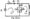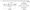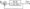# Control System June 2010

Note: Answer any FIVE full questions,

selecting at least TWO questions from each part,

PART-A

1 a. Distinguish between open loop and closed loop control systems. Give two examples for each.

b.  Write the differential equations for the mechanical rotational system shown in Fig. Ql(b) below. Obtain the torque-current analogy of the system. List all the analogous quantities.Fig. Q (b)C. The force-voltage analogy of a mechanical translational system is given in Fig.Q. 1(c). Obtain its analogous mechanical system.b. Determine the gain Xg/ Xi, of the system described by the signal flow graph given in Fig.Q.2 (b).

3 a. Derive an expression for the unit step response of an underdamped second order system, with usual notations. Sketch this response and mark clearly the rise time tr, peak time tp, settling time U and peak overshoot Mp.

b.  A unity feedback control system is characterized by an open loop T.F, G(s)=s(s + a) Where K and a are positive constants.

i)    By what factor the amplifier gain K should be reduced so that the peak overshoot of the unit step response reduces from 75% to 25%?

ii)   Find the values of K and a, so that, damping ratio is 0.6 and frequency of damped oscillations is 8 rad / sec. Also find the peak value of the response, when the system is excited by a step of 2V.

iii)  If the above open loop T.F. G(s) is multiplied with a factor (s+p), and the closed loop poles are located at -1 ± jl, find the values of K, a and p so that the steady state value of error for a ramp input equals 1/10.

4 a. Determine the values of k and a, so that the system shown in Fig.Q.4(a), oscillates at a frequency of 2 rad / sec.Fig.Q.4(a)

b. The open loop T.F. of a unity feedback control system is given by K.

GS‘” (s + 2Xs + 4)(S2 + 6s + 25)’

(c)Determine the range of values of K for system stability. What is the value of K which gives sustained oscillations? What is the oscillation frequency?

PART-2

5 a. ketch the root locus diagram for a unit feedback control system with G(s) = -r-=W s(s +8s + 17) using the rules of construction and by determining the break away /break in points and the angle of departure.

(b) Find the value of K for which the system just oscillates. From the root locus, determine the value of K for a damping ratio of 0.5.

6 a. State and explain Nyquist stability criteria.

b.   Discuss the stability of the unity feedback control system with G(s) = , .

s (1 + sj Nyquist criteria. If H(s) = 1 + 2s, test the stability of the system.

7  a. A unity feedback control system is characterized by an open loop transfer function G(s)h(s) = r. Using Bode plots, find : s(s + lX0.1s + l)

i)  The value of K to a give gain margin of 10 dB

ii)    Value of K to give a phase margin of 24°.

b.  Find the T.F of the system whose Bode diagram is given in Fig. Q7(b)8  a.Define state variable and state transition matrix. List the properties of the state transition matrix.

b.  Obtain the state model for the electrical system given in Fig. Q 8(b), choosing the state variables as ii(t), i2(t) and vc(t).